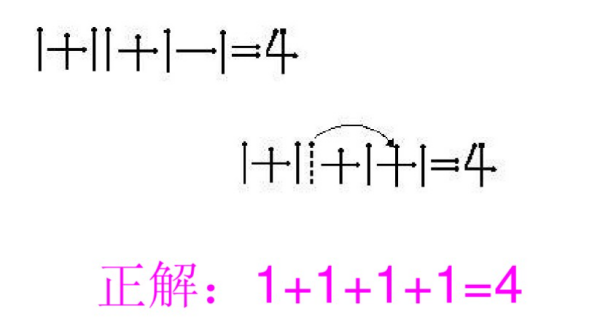# 1+7=1移动一根火柴变成另一个等式怎么变？

• A+

1+7=1→”1+1≠1“；移动数字7中的一根火柴构成不等号。1+7=1→“1±1=1”；移动数字7中的一根火柴构成”±“号。

### 问题内容

1+7=1移动一根火柴变成另一个等式怎么变？ 仅考虑改变等式而不考虑等式的正确性的话有以下几种改法：

1+7=1→”1+1≠1“；移动数字7中的一根火柴构成不等号。

1+7=1→“1±1=1”；移动数字7中的一根火柴构成”±“号。

1+7=1→“1-7≠1”；移动“+”中的一根火柴使之变为减号以及构成不等号。

1+7=1→“1-7=11”；如上移动加号构成数字“11”。

### 网友回答

1+7=1→”1+1≠1“；移动数字7中的一根火柴构成不等号。

1+7=1→“1±1=1”；移动数字7中的一根火柴构成”±“号。

1+7=1→“1-7≠1”；移动“+”中的一根火柴使之变为减号以及构成不等号。

1+7=1→“1-7=11”；如上移动加号构成数字“11”。